In a previous article, I mentioned Best Open Source Database Software (DBMS) but you must have heard of functions like AVG, Count etc in SQL or SQL server, but many of us still don't know how to use Aggregate functions in SQL, so in this guide, I am going to explain all of the Aggregate functions (SUM, COUNT, AVG, MIN, MAX) in SQL server with an example.

## What are Aggregate Functions in SQL?

An aggregate function is a function where the values of multiple rows are grouped together to form a single value of more significant meaning or measurements such as a set or a list.

So, in other words, Aggregate functions perform a calculation on a set of values and return a single value.

The aggregate functions – `COUNT`, `SUM`, `AVG`, `MAX`, `MIN`  – don't handle `NULL` in the same way as ordinary functions and operators. Instead of returning `NULL` as soon as a `NULL` operand is encountered, they only take non-`NULL` fields into consideration while computing the outcome

SQL Aggregate functions are among the ones most widely used in reporting & data joining scenarios. Here are the few SQL Aggregate functions which I am going to explain today

1. COUNT ()  :  returns the total number of values in a given column
2. SUM ()  : returns the sum of the numeric values in a given column
3. AVG  () : returns the average value of a given column
4. MIN() : returns the smallest value in a given column
5. MAX () : returns the largest value in a given column

Let's discuss each of them with an example. I will be using sample database "AdventureWorks" on my local SQL server database, you can  download and install the complete AdventureWorks Database if you have an SQL server, else you can download SQL server various version first.

Let's create a new table on which using which I will be explaining our example:

``````USE [AdventureWorks2012]
GO

SELECT
P.BusinessEntityID AS EmpID, P.Title, P.FirstName, P.MiddleName, P.LastName,
E.Gender, E.MaritalStatus, E.BirthDate AS DOB, E.HireDate AS DOJ, E.JobTitle,
D.Name AS DeptName, S.Rate
INTO dbo.Employee -- Create a new table here and load query data
FROM [Person].[Person] P
INNER JOIN [HumanResources].[Employee] E
CROSS APPLY (
SELECT TOP 1 DepartmentID
FROM [HumanResources].[EmployeeDepartmentHistory] DH
ORDER BY StartDate DESC) EDH
INNER JOIN [HumanResources].[Department] D
ON D.DepartmentID = EDH.DepartmentID
INNER JOIN [HumanResources].[EmployeePayHistory] S

SELECT * FROM dbo.Employee
GO``````

Output will be a list of Employee's### COUNT() function

Count function is used to return the number of rows in a table or a group and returns an INT (integer).

Syntax

``````-- Syntax for SQL Server and Azure SQL Database

COUNT ( { [ [ ALL | DISTINCT ] expression ] | * } )
[ OVER (
[ partition_by_clause ]
[ order_by_clause ]
[ ROW_or_RANGE_clause ]
) ]  ``````

Let's try to use the COUNT function in the above-created table in SQL server with different scenario's

``````-- To get count of all reords in a table:
select COUNT(*) from dbo.Employee -- 316

-- Within Group: get count of records having Gender = 'M':
select COUNT(*) from dbo.Employee WHERE Gender = 'M' -- 228

-- Using ColumnName to COUNT:
select COUNT(FirstName) from dbo.Employee -- 316

-- Using DISTINCT with COUNT:
select COUNT(DISTINCT FirstName) from dbo.Employee -- 224

-- Using Count to provide output column a name
select COUNT(DISTINCT FirstName) as Total from dbo.Employee  -- 224``````

I have commented out each of the cases so you can understand the difference, let's take a look on the output, when it is executed in SQL serverThe Last example which I have highlighted using red border show's the output as custom named, column, so basically, you can provide your own name to the output of COUNT and can use it in other sub-query also.

Using COUNT() function with GROUP BY

``````-- Get count of Employees in every Department:
select
DeptName,
COUNT(*) as EmpCount
from dbo.Employee
GROUP BY DeptName``````### AVG Aggregate function

Avg function returns the average of the values in a group. Null values are ignored.

AVG () computes the average of a set of values by dividing the sum of those values by the count of nonnull values. If the sum exceeds the maximum value for the data type of the return value an error will be returned.

AVG is a deterministic function when used without the OVER and ORDER BY clauses. It is non-deterministic when specified with the OVER and ORDER BY clauses.

Syntax

``````AVG ( [ ALL | DISTINCT ] expression )
OVER ( [ partition_by_clause ] order_by_clause )``````

Example for using AVG with Group By, the following example produces summary values for each sale territory in the AdventureWorks2012 database. The summary lists the average bonus received by the sales people in each territory.

``````SELECT TerritoryID, AVG(Bonus)as 'Average bonus'
FROM Sales.SalesPerson
GROUP BY TerritoryID;
GO  ``````

Output:### MIN() , MAX() functions

MIN() & MAX() functions are used to returns the Minimum & Maximum values from a table or a group, respectively.

Syntax for Min

``````MIN ( [ ALL | DISTINCT ] expression )
OVER ( [ partition_by_clause ] order_by_clause )  ``````

Syntax for Max

``````MAX ( [ ALL | DISTINCT ] expression )
OVER ( [ partition_by_clause ] order_by_clause )     ``````

MAX & MIN ignores any null values.

For character columns, MAX finds the highest value in the collating sequence while MIN finds the value that is lowest in the sort sequence.

Let's take a look at some example, following example returns the lowest (minimum) tax rate. The example uses the AdventureWorks2012 database

``````SELECT MIN(TaxRate)
FROM Sales.SalesTaxRate;
GO  ``````

Output

``5.00``

For MAX

``````SELECT MAX(TaxRate)
FROM Sales.SalesTaxRate;
GO ``````

Output

``19.60``

Using Min, Max, AVG, COUNT all together

``````SELECT DISTINCT Name
, MIN(Rate) OVER (PARTITION BY edh.DepartmentID) AS MinSalary
, MAX(Rate) OVER (PARTITION BY edh.DepartmentID) AS MaxSalary
, AVG(Rate) OVER (PARTITION BY edh.DepartmentID) AS AvgSalary
,COUNT(edh.BusinessEntityID) OVER (PARTITION BY edh.DepartmentID) AS EmployeesPerDept
FROM HumanResources.EmployeePayHistory AS eph
JOIN HumanResources.EmployeeDepartmentHistory AS edh
JOIN HumanResources.Department AS d
ON d.DepartmentID = edh.DepartmentID
WHERE edh.EndDate IS NULL
ORDER BY Name;  ``````The above example uses MIN, MAX, AVG, and COUNT functions with the OVER clause to provide aggregated values for each department in the HumanResources.Department table in the AdventureWorks2012 database.

### SUM() function

It returns the sum of all the values, or only the DISTINCT values, in the expression. SUM can be used with numeric columns only. Null values are ignored.

``````SUM ( [ ALL | DISTINCT ] expression )
OVER ( [ partition_by_clause ] order_by_clause )   ``````

For example, the below query shows, using the SUM function to return summary data in the AdventureWorks2012 database.

``````SELECT Color, SUM(ListPrice), SUM(StandardCost)
FROM Production.Product
WHERE Color IS NOT NULL
AND ListPrice != 0.00
AND Name LIKE 'Mountain%'
GROUP BY Color
ORDER BY Color;
GO  ``````

Output### Grouping() function in SQL server

Grouping indicates whether a specified column expression in a GROUP BY list is aggregated or not. It returns 1 for aggregated or 0 for not aggregated in the result set.

Syntax

``GROUPING ( <column_expression> )  ``

In the following example groups `SalesQuota` and aggregates `SaleYTD` amounts in the AdventureWorks2012 database. The GROUPING function is applied to the SalesQuota column.

``````SELECT SalesQuota, SUM(SalesYTD) 'TotalSalesYTD', GROUPING(SalesQuota) AS 'Grouping'
FROM Sales.SalesPerson
GROUP BY SalesQuota WITH ROLLUP;
GO  ``````

Here is the screenshot of output.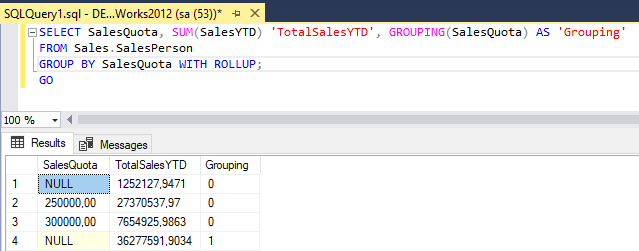### CheckSum in SQL server

It is used to build Hash indexes. The `CHECKSUM` function returns the checksum value computed over a table row, or over an expression list.

Syntax

``CHECKSUM ( * | expression [ ,...n ] )  ``

Where * can be anything like text, this argument specifies that the checksum computation covers all table columns.

` CHECKSUM` returns an error if any column has a noncomparable data type. Noncomparable data type includes data like text, XML, image, cursor etc.

Example

``````USE AdventureWorks2012;
GO
ALTER TABLE Production.Product
GO
CREATE INDEX Pname_index ON Production.Product (cs_Pname);
GO  ``````

The above query, adds a computed checksum column to the table we want to index. It then builds an index on the checksum column.

After executing above query, if you will check updated table "`[AdventureWorks2012].[Production].[Product]`', you will find a new column "cs_pname"

### CheckSum_AGG() in SQL server

The same as `CHECKSUM`, but the primary difference is that `CHECKSUM` is oriented around rows, whereas `CHECKSUM_AGG` is oriented around columns.

This function returns the checksum of the values in a group. `CHECKSUM_AGG` ignores null values.

Syntax

``CHECKSUM_AGG ( [ ALL | DISTINCT ] expression )  ``

Where, When used:

ALL
Applies the aggregate function to all values. ALL is the default argument.

DISTINCT
Specifies that CHECKSUM_AGG returns the checksum of unique values.

expression
An integer expression. CHECKSUM_AGG does not allow use of aggregate functions or subqueries.

Example:

``````--Get the checksum value before the column value is changed.

SELECT CHECKSUM_AGG(CAST(Quantity AS int))
FROM Production.ProductInventory;
GO  ``````

Output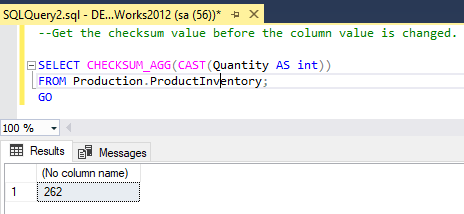### Count_Big() function

This function returns the number of items in a group. The data type returned is of type bigint.

Syntax

``COUNT_BIG ( { [ [ ALL | DISTINCT ] expression ] | * } )  ``

Example

``````SELECT COUNT_BIG (*)
FROM HumanResources.Employee;
GO  ``````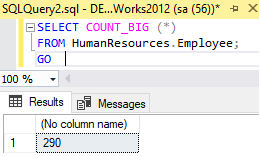COUNT_BIG(*) returns the number of items in a group. This includes NULL values and duplicates.

COUNT_BIG (ALL expression) evaluates expression for each row in a group, and returns the number of nonnull values.

COUNT_BIG (DISTINCT expression) evaluates expression for each row in a group, and returns the number of unique, nonnull values.

### STDEV() function

It returns the standard deviation of all values in expression. Stdev ignores any NULL values.

Syntax

``STDEV( <expression> )``

Example

``````SELECT STDEV(Bonus)
FROM Sales.SalesPerson;
GO  ``````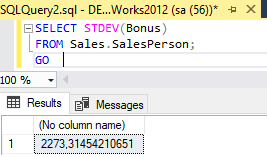### STDDEVP() function

It returns the standard deviation for the population of all values in expression. Stdevp ignores any NULL values.

Syntax

``STDEVP ( [ ALL | DISTINCT ] expression )  ``

Example

``````SELECT STDEVP(Bonus)
FROM Sales.SalesPerson;
GO  ``````

Output:

``````---No Column Name---
2205,43905349595``````

### VAR() Function

Returns the variance of all values in expression. Var ignores any NULL values.

Syntax

``VAR ( [ ALL | DISTINCT ] expression )  ``

Example

``````SELECT VAR(Bonus)
FROM Sales.SalesPerson;
GO  ``````

Output:

``````--no Column Name--
5167959,00735294``````

Above example returns the variance for all bonus values in the SalesPerson table in the AdventureWorks2012 database.

### VARP() function

It returns the statistical variance for the population for all values in the specified expression.

Syntax

``VARP ( [ ALL | DISTINCT ] expression )  ``

Example

``````SELECT VARP(Bonus)
FROM Sales.SalesPerson;
GO  ``````

Output

``````--No Column Name--
4863961,41868512``````

Above example returns the variance for the population for all bonus values in the SalesPerson table in the AdventureWorks2012 database.

### Grouping_Id() function

It is a function, that computes the level of grouping. GROUPING_ID can be used only in the SELECT <select> list, HAVING, or ORDER BY clauses when GROUP BY is specified.

Syntax:

``GROUPING_ID ( <column_expression>[ ,...n ] )  ``

The GROUPING_ID <column_expression> must exactly match the expression in the GROUP BY list.

Example

``````SELECT D.Name
,CASE
WHEN GROUPING_ID(D.Name, E.JobTitle) = 0 THEN E.JobTitle
WHEN GROUPING_ID(D.Name, E.JobTitle) = 1 THEN N'Total: ' + D.Name
WHEN GROUPING_ID(D.Name, E.JobTitle) = 3 THEN N'Company Total:'
ELSE N'Unknown'
END AS N'Job Title'
FROM HumanResources.Employee E
INNER JOIN HumanResources.EmployeeDepartmentHistory DH
INNER JOIN HumanResources.Department D
ON D.DepartmentID = DH.DepartmentID
WHERE DH.EndDate IS NULL
AND D.DepartmentID IN (12,14)
GROUP BY ROLLUP(D.Name, E.JobTitle);  ``````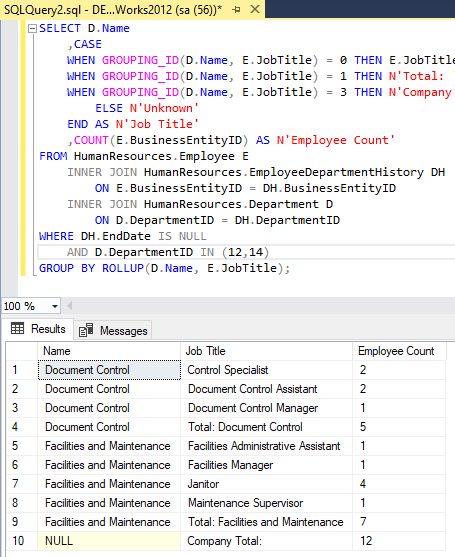The above example, returns the count of employees by `Name` and `Title, Name,` and company total in the AdventureWorks2012 database. GROUPING_ID() is used to create a value for each row in the Title column that identifies its level of aggregation.

That's it, these are the all aggregate functions available in SQL server.

You may also like to read:

TRIM(), LTRIM(), RTRIM() Functions in SQL Server

Get month and year in sql server from date

Get Date From Datetime in SQL Server

How to check sql server version? (Various ways explained)

SQL Server Management Studio (SSMS) Alternatives (Mac, Windows, Linux)

Microsoft SQL Server Versions List

How to backup SQL Server database ? (Various Ways explained)

How to Create database in sql server management studio (With Insert/Delete)